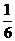Chapter 10, Problem 82AP### Introductory Chemistry: A Foundati...

9th Edition
Steven S. Zumdahl + 1 other
ISBN: 9781337399425

#### Solutions

Chapter
Section### Introductory Chemistry: A Foundati...

9th Edition
Steven S. Zumdahl + 1 other
ISBN: 9781337399425
Textbook Problem
1 views

# Consider the following equations:mg src=Images/HTML_99425-10-82AP_image001.jpg alt="" align="top"/>ppose the first equation is reversed and multiplied bythe second and third equations are divided by 2, and the three adjusted equations arc added. What is the net reaction and what is the overall heat of this reaction?

Interpretation Introduction

Interpretation:

The net reaction of the given three equations should be determined if first equation is reversed and multiplied by 1/6, second and third equations are divided by 2 and all the three equation are added.

Concept Introduction:

Enthalpy of a reaction is a state function thus; change in enthalpy of a reaction does not depend on the path of the reaction.

The change in enthalpy is same if the same reaction takes place in one step or series of steps. This is known as Hess’s law. There are following characteristics of change in enthalpy which are important to calculate the change in enthalpy of a reaction using Hess’s law.

1. For a reversed reaction, the sign of enthalpy change of the reaction also reversed.
2. If any reactant or product is multiplied by any integer in the chemical reaction, enthalpy change of that reactant or product is also multiplied by the same integer.
Explanation

Given Information:

The following reactions are given:

3A+6B3D     ΔH=403 kJ/molE+2FA     ΔH=105.2 kJ/molCE+3D      ΔH=+64.8 kJ/mol

Calculation:

On reversing the first reaction,

3D3A+6B        ΔH=+403 kJ/mol

Now, multiplying it by 1/6,

16(3D3A+6B)        ΔH=16(+403 kJ/mol)

Thus,

12D12A+B             ΔH=+67.167 kJ/mol...... (1)

Dividing, second and third reaction by 2:

12(E+2FA)     ΔH=12(105.2 kJ/mol)

Or,

12E+F12A    ΔH=52

### Still sussing out bartleby?

Check out a sample textbook solution.

See a sample solution

#### The Solution to Your Study Problems

Bartleby provides explanations to thousands of textbook problems written by our experts, many with advanced degrees!

Get Started